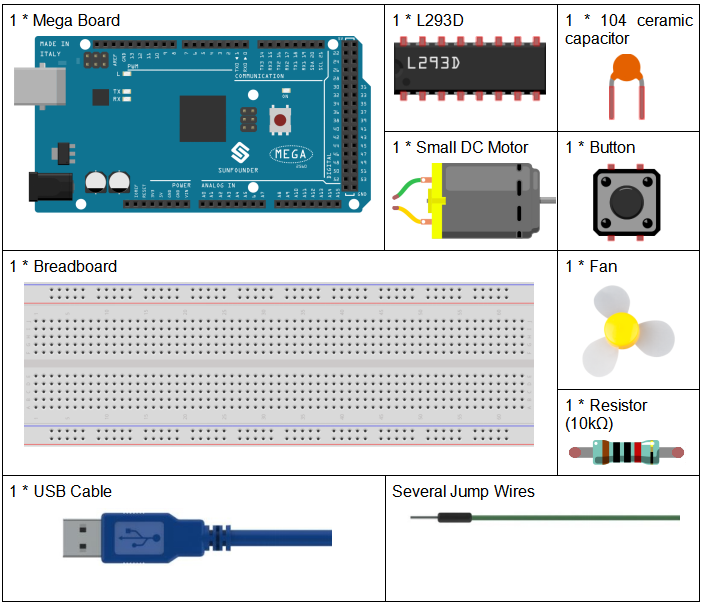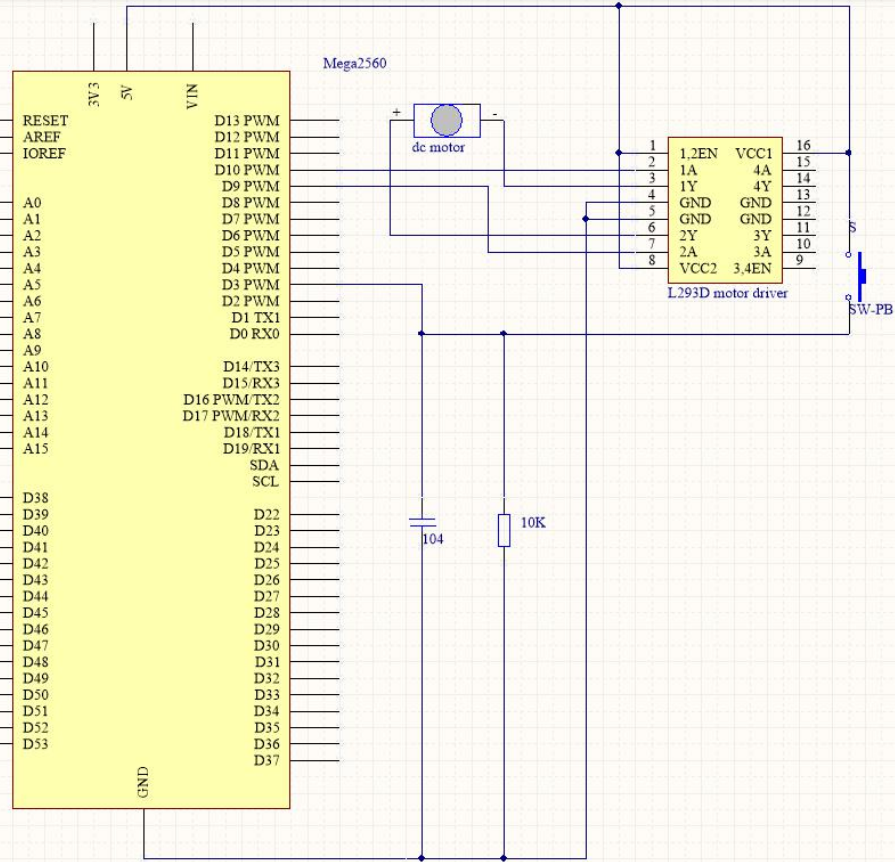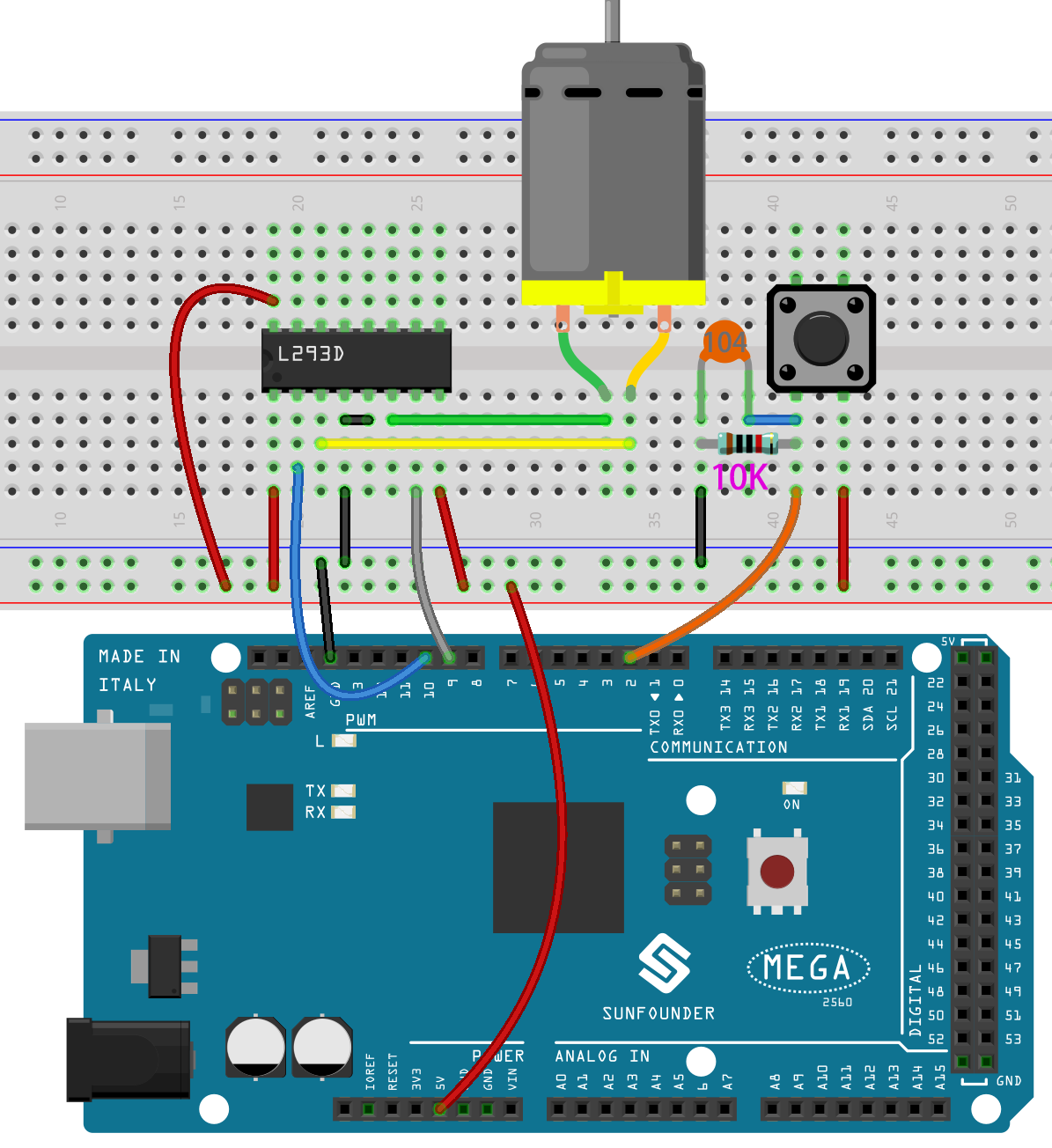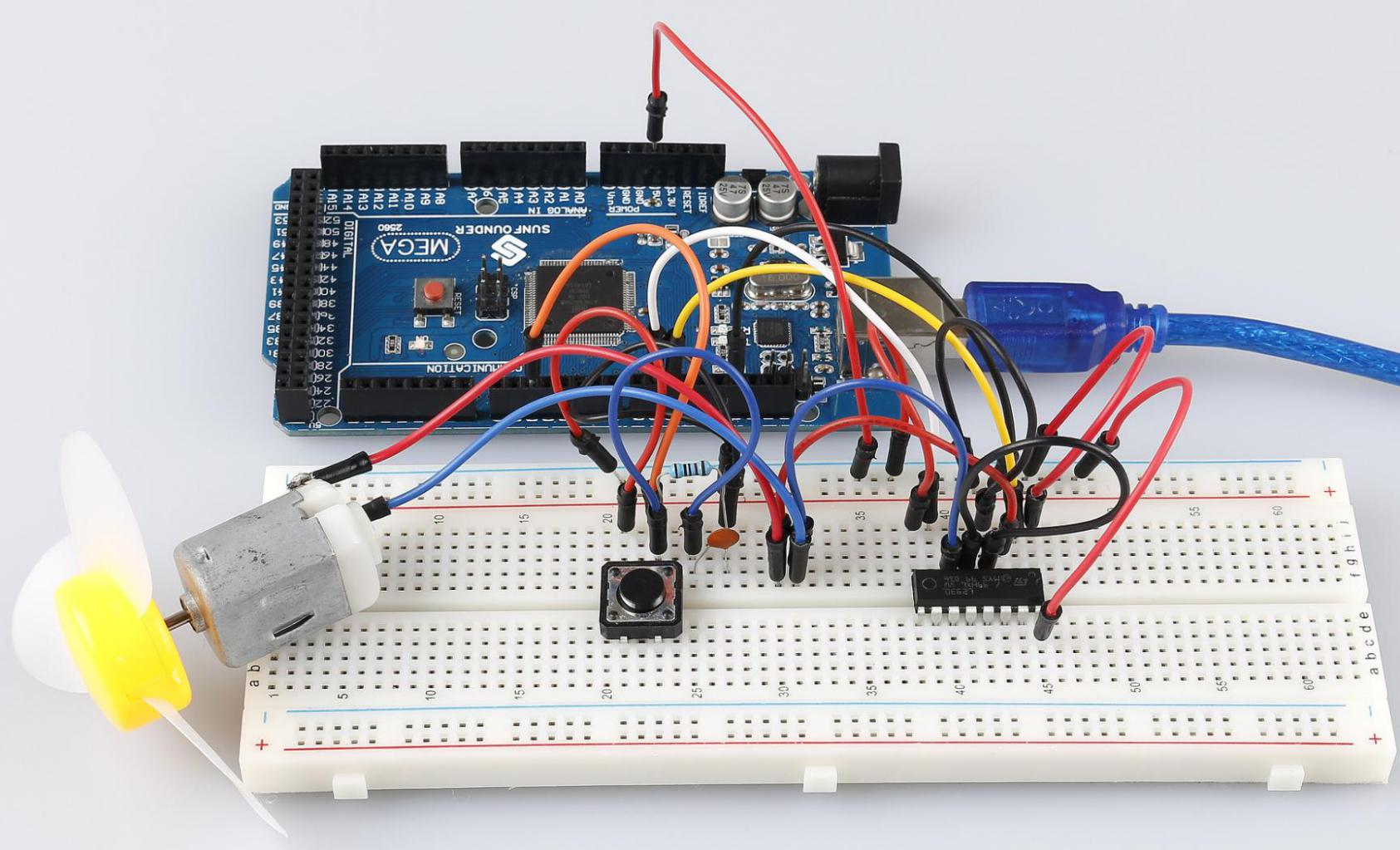# Lesson 22 Simple Creation-Small Fan¶

## Introduction¶

In this experiment, we will learn how to control the direction and speed of a small-sized DC motor by a driver chip L293D. Making simple experiments, we will just make the motor rotate left and right, and accelerate or decelerate automatically.

## Components¶## Schematic Diagram¶

The maximum current of an Arduino I/O port is 20mA but the drive current of a motor is at least 70mA. Therefore, we cannot directly use the I/O port to drive the current; instead, we can use an L293D to drive the motor.

The Enable pin 1,2EN of the L293D are connected to 5V already, so L293D is always in the working state. Connect pin 1A and 2A to pin 9 and 10 of the control board respectively. The two pins of the motor are connected to pin 1Y and 2Y respectively. When pin 10 is set as High level and pin 9 as Low, the motor will start to rotate towards one direction. When the pin 10 is Low and pin 9 is High, it rotates in the opposite direction.

The schematic diagram:## Experimental Procedures¶

Step 1: Build the circuitStep 2: Open the code file.

Step 3: Select the Board and Port.

Step 4: Upload the sketch to the board.

The blade of the DC motor will begin rotating left and right, in a speed that varies accordingly.Code

## Code Analysis¶

This code nests five `if` statements to determine the button press status.

• The first if statement is used to determine if the button is pressed.

• The second if statement is used to determine if 50ms has elapsed.

• The third if statement is used to determine if the button has been pressed after 50ms, so as to avoid false touches.

• The fourth if statement is used to record the number of button presses, adding 1 to `stat` for each press.

• The fifth if statement is used to determine if the number of button presses is greater than 4. If so, `stat` is cleared to zero.

switch() statement

```switch(stat)
{
case 1:
clockwise(rank1);// When stat=1, set the rotate speed of the motor as rank1=150
break;
case 2:
clockwise(rank2);// When stat=2, set the rotate speed of the motor as rank1=200
break;
case 3:
clockwise(rank3);// When stat=3, set the rotate speed of the motor as rank1=250
break;
default:
clockwise(0);
}
```

The `switch` statement, like the `if` statement, `switch case` allows the programmer to control the flow of the program with different code executed under various conditions. In particular, the switch statement compares the value of a variable with the value specified in the case statement. When a case statement is found whose value matches the value of a variable, the code in that case statement is run. If there is no `break` statement, the `switch` statement will continue to execute the following expression until `break` or until it reaches the end of the switch statement.

In this part of the code.

• If stat = 1, let the fan rotate at speed rank1(150).

• If stat = 1, let the fan rotate at speed rank2(200).

• If stat = 1, let the fan rotate at speed rank3(250).

• If stat = 0, let the fan rotate at speed 0.

clockwise() function

```void clockwise(int Speed)//
{
analogWrite(motorIn1,0);
analogWrite(motorIn2,Speed);
}
```

This function sets the speed of the motor: write `Speed` to pin 9 and 0 to pin 10. The motor rotates in a certain direction with the value of `Speed`.

## Experiment Summary¶

In this experiment, you can also control the motor to rotate or not. Just connect pin 1, 2EN of the L293D to an I/O port of the control board. Set 1, 2EN as High level, and the motor will start rotating; set it as Low level, it will stop the rotating.